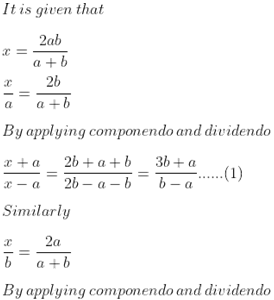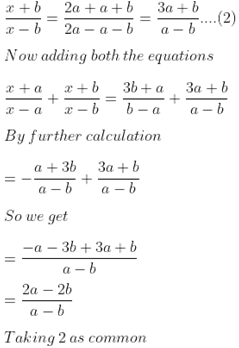Guru

# If x=2ab/(a+b) find the value of x+a/x−a+x+b/x−b

• 0

This is very important and famous question of this pattern, and has been asked in exam many a time

Question no. 7 From ML aggarwal book, class10, chapter 7, ratio and proportion

We have been given that

x=2ab/(a+b)

And we have to find the value of x+a/x−a+x+b/x−b

Ques 7, 7.3

Share